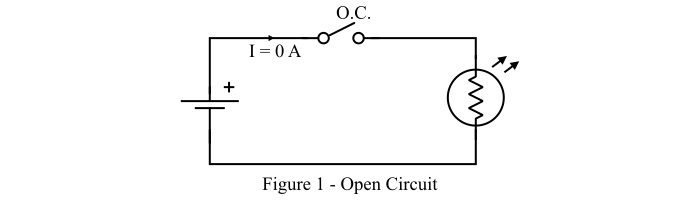# Difference between Open Circuit and Closed Circuit

An electric circuit or simply circuit is an arrangement of circuit components such as energy sources, resistors, inductors, capacitors, etc. which allows the flow of electric current from source to load.

Based on the ON & OFF condition of the circuit, it can be of two types viz −

• Open Circuit

• Closed Circuit

In this article, we will discuss the key differences between open and closed circuits. But, before discussing the difference, let's get a basic overview of what open and closed circuits are, which will help in understanding their differences better.

## What is an Open Circuit?

When there is a break or gap in an electric circuit which disallow the flow of current through the circuit is known as open circuit.In an open circuit, no current can flow from the source to the load due to incomplete path between the energy source and the load. Therefore, the open circuit represents the circuit in OFF state. The open circuit may be due to switch in OFF state or failure of any component in the circuit. A simple open circuit is shown in Figure-1.

## What is a Closed Circuit?

An electric circuit is said to be closed if there is continuous path from the energy source to the load. Thus, an electric circuit which has a closed loop path to flow of current from source to load is called a closed circuit.

The closed circuit represents the ON state of the circuit. The main parts of a closed circuit are: a conducting wire, source of energy (ex. battery) and an electric load. These components joined together to form a closed loop so that current can flow from source to load through wire.A simple closed circuit is shown in Figure-2, where a battery is connected to a lamp through the wires and a switch. The closed switch completes the path to flow the current in the circuit.

## Difference between Open Circuit and Closed Circuit

Open and close are the two states of an electric circuit. However, there are many differences between open circuit and closed circuit that are highlighted in the following table

Basis of Difference Open Circuit Closed Circuit
Definition An electric circuit in which there is a gap or break and disallows the flow of current is called an open circuit. An electric circuit which has a closed loop through which current can flow is called a closed circuit.
State of circuit Open circuit represents the OFF state of the circuit. Closed circuit represents the ON state of the circuit.
Path An open circuit is a discontinuous path. A closed circuit is continuous path.
Continuity There is no continuity in the open circuit. The closed circuit has continuity.
Electric current In open circuit, the electric current cannot flow in the circuit, i.e. current is zero. A definite current flows in the closed circuit.
Voltage In an open circuit, the entire supply voltage appears across the open terminals of the circuit. In a closed circuit, the supply voltage distributed across the loads depending the load parameters.
Circuit resistance The resistance of an open circuit is ideal infinite, but practically very high. The resistance of the closed circuit is relatively less than open circuit and is decided by the circuit elements such as resistance of wires, elements, etc.
Examples A common example of an open circuit is the circuit of lamp with switch in OFF state. The example of a closed switch is the circuit of lamp with switch in ON state.

## Conclusion

The most significant difference between an open circuit and a closed circuit is that an open circuit is a discontinuous path that restricts the flow of electricity from source to load, while a closed circuit is a continuous path which allows the flow of electricity from source to load. However, both open circuit and closed circuit may be the two states of the same circuit that can be achieved by turning the switch ON and OFF.